# Euler's Totient Function

##### Age 16 to 18Challenge Level

Question 1: Show that $\phi(15) = 8$.

Rayn, Torsten, Archie, Rupert, Jack, Takumi and Joe from Abingdon, Nayanika from The Tiffin Girls' School, Wiktor from LAE Tottenham, Mohsin from Oasis Academy Hadley and Olivia from Roedean, all in the UK, Ella from Jersey College for Girls in Jersey and Mahdi from Mahatma Gandhi International School in India all found $\phi(15).$ This is Ella's work: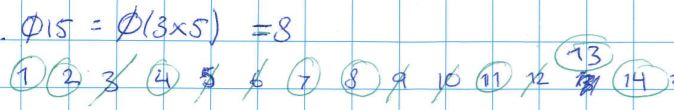Nayanika showed how to find $\phi(15)$ in a video which also introduces a different famous $\phi$ in mathematics: the golden ratio. Although they are written using the same letter, the golden ratio and Euler's Totient function are not directly related. Click here to see Nayanika's video.

Question 2: Investigate $\phi(p)$ where $p$ is a prime number.  Can you find a general expression for $\phi(p)$?

Rayn, Torsten, Archie, Rupert, Jack, Takumi and Joe, Nayanika, Ella, Wiktor, Mohsin, Mahdi and Olivia found an expression for $\phi (p)$. Rayn, Torsten, Archie, Rupert, Jack, Takumi and Joe sent these examples: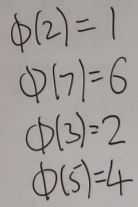This is Mohsin's work: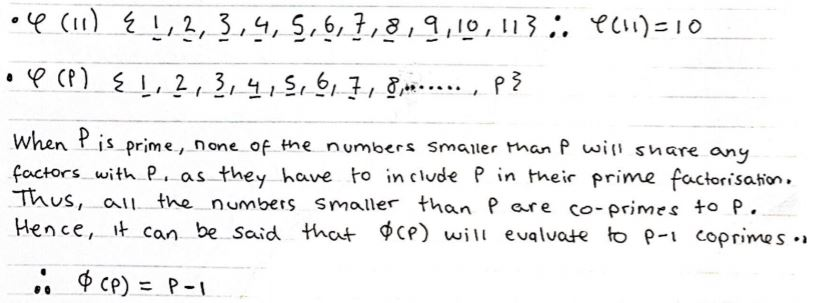Question 3: Investigate $\phi(2^n)$ where $n=1, 2, 3, ...$.  Can you find a general expression for $\phi(2^n)$?  What about $\phi(3^n)$?

Rayn, Torsten, Archie, Rupert, Jack, Takumi and Joe, Wiktor, Mahdi, Olivia and Mohsin tried some examples and found a general expression for $\phi (p^n).$ This is Mahdi's work for $\phi(2^n)$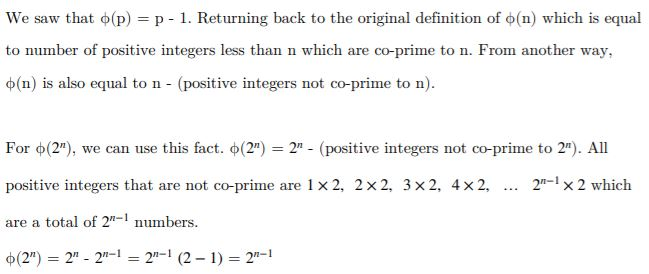This is Olivia's work for $\phi (3^n)$ and $\phi (p^n)$: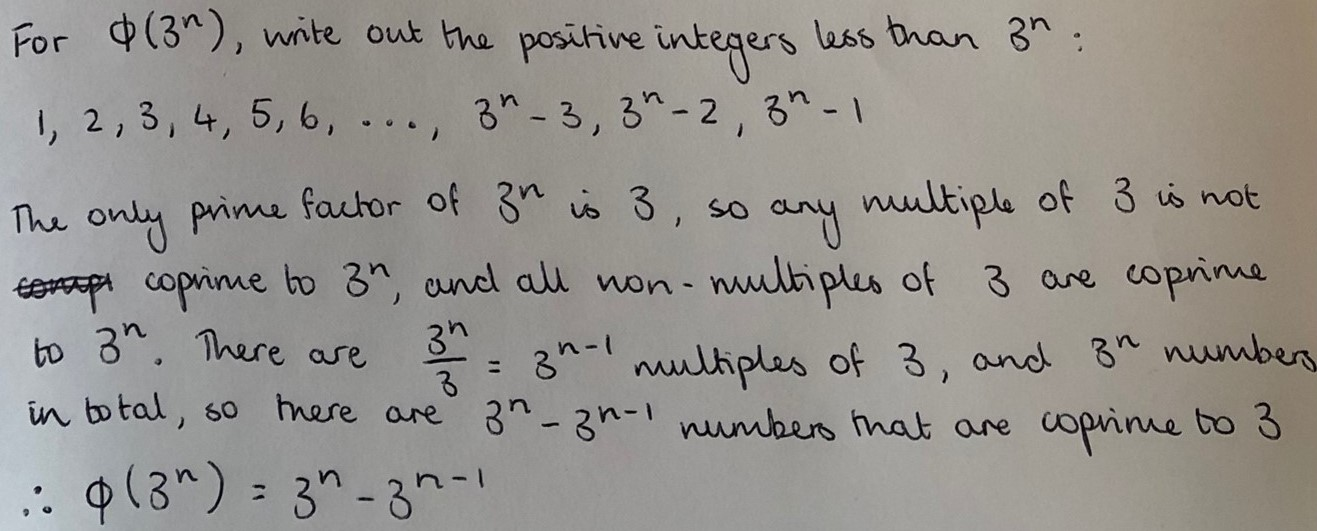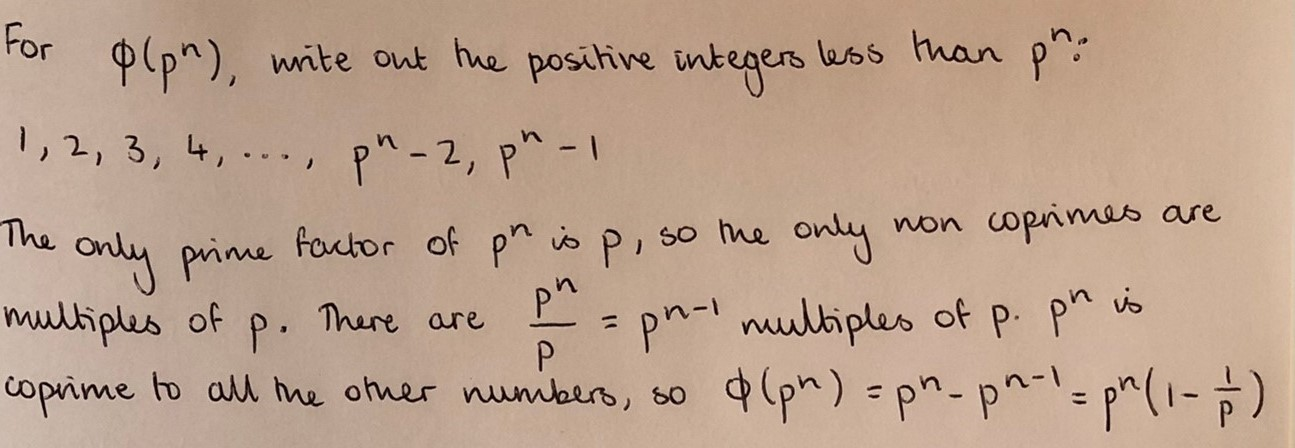Question 4: Under which conditions is $\phi(nm) = \phi(n) \times \phi(m)$ true?

Nayanika and Ella said that $\phi(nm) = \phi(n) \times \phi(m)$ when $m$ and $n$ are prime. This is Ella's work: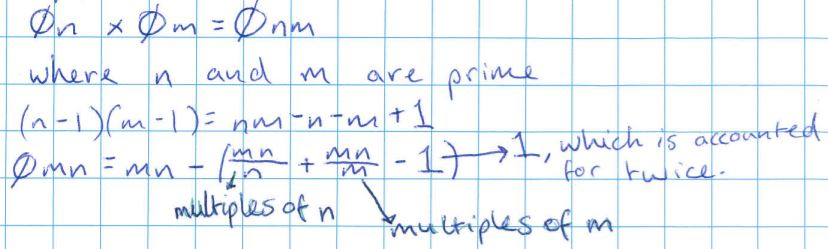Rayn, Torsten, Archie, Rupert, Jack, Takumi and Joe, Ella, Wiktor, Mohsin, Mahdi and Olivia found that it sometimes works when $m$ and $n$ are not prime. This is Olivia's work, with some notes added to the diagram to support some of the algebra: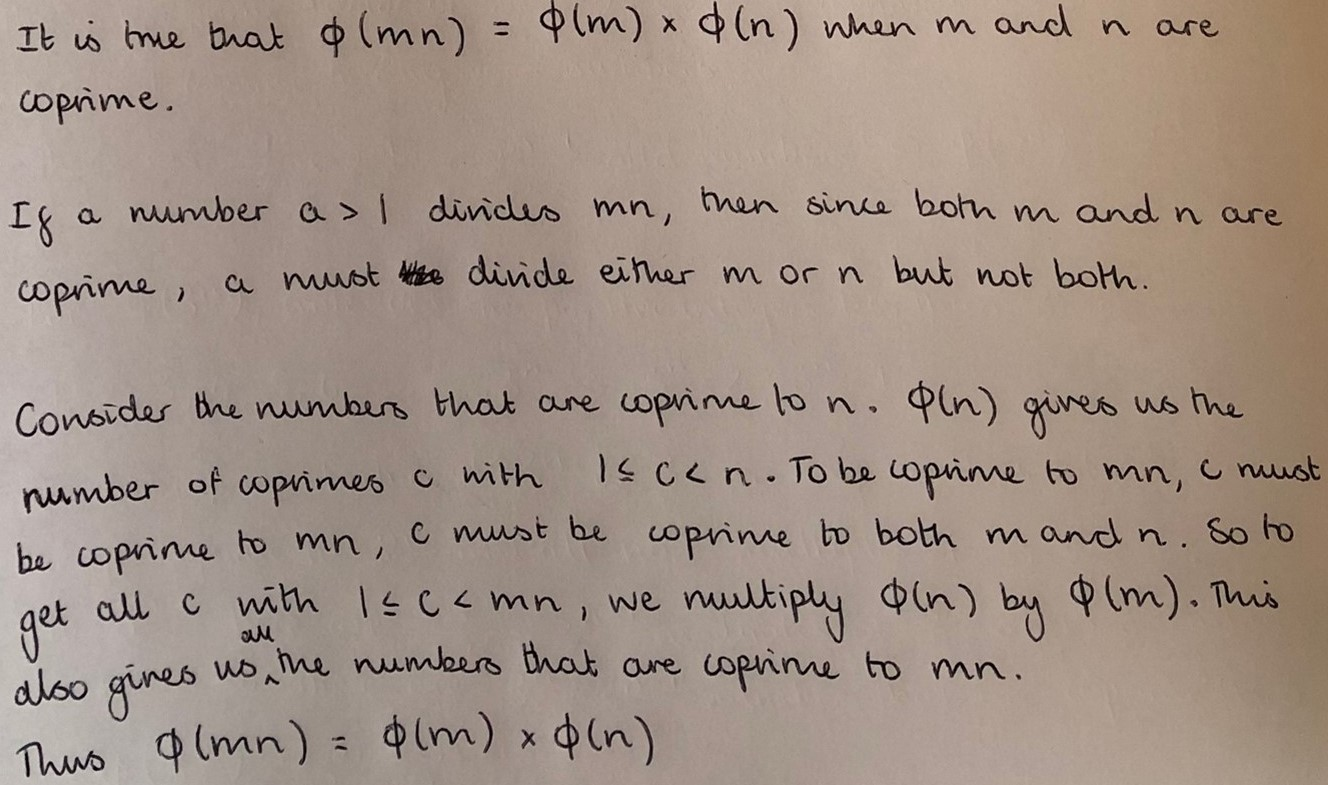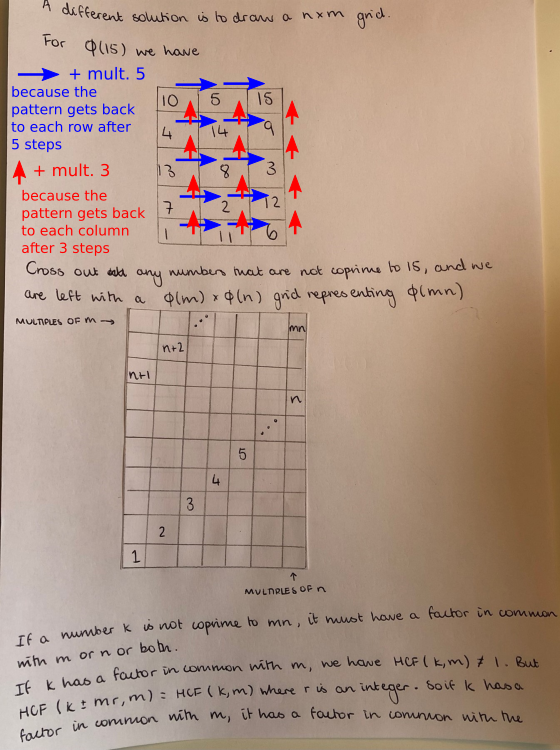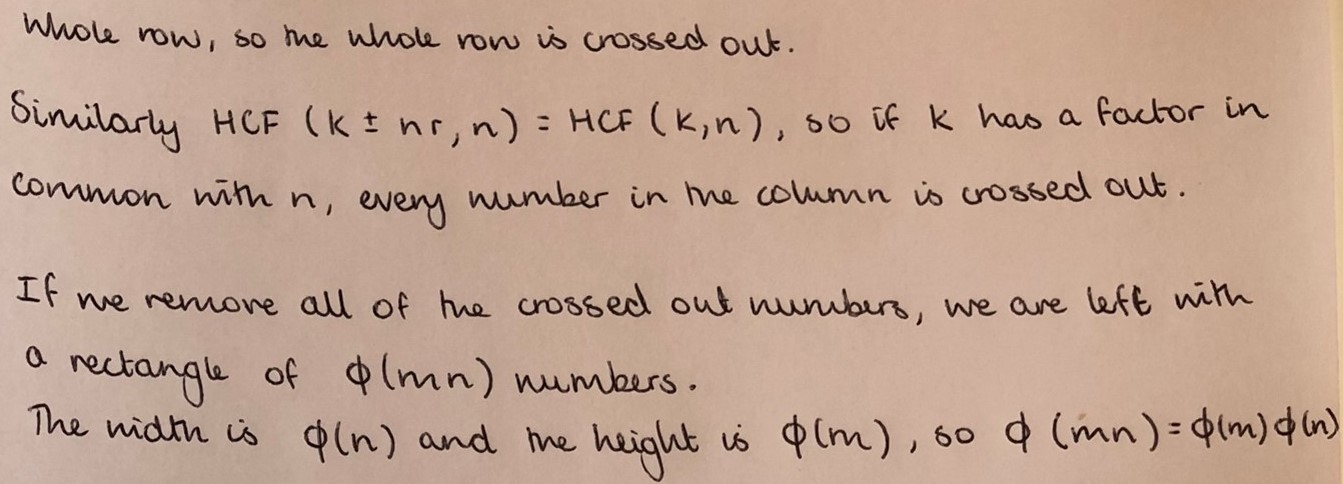Question 5: Can you find a general expression for $\phi(n)$?

Rayn, Torsten, Archie, Rupert, Jack, Takumi and Joe and Ella thought about using prime factorisations. Wiktor, Mohsin, Mahdi and Olivia found an expression for $\phi(n)$ using the prime factorisation of $n.$ This is Wiktor's work: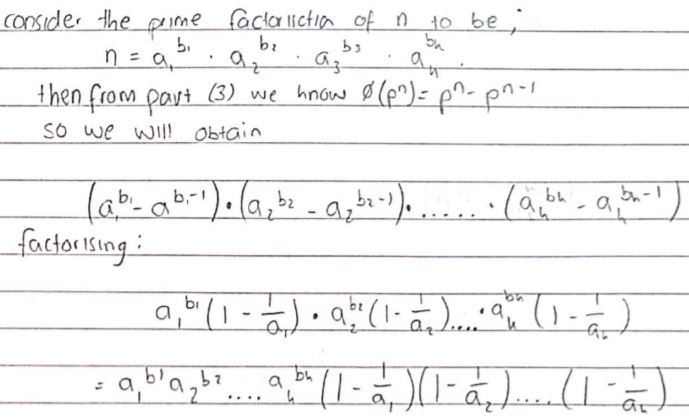which is equal to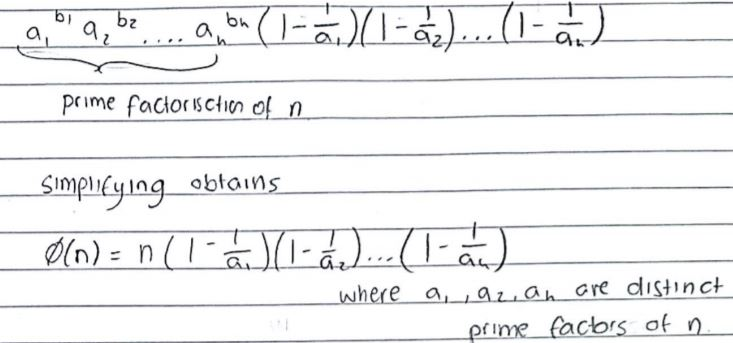Rayn, Torsten, Archie, Rupert, Jack, Takumi and Joe wrote a python program which computes Euler's Totient Function:

def totient (x):
ans=1
list=[]
list2=[]
counter=0
while x != 1:
for i in range (2,x+1):
if x%i==0:
x=x//i
if i not in list:
list.append(i)
else:
if counter!=i:
list2.append(i)
counter=i
list2.append(i)
break
for i in list2:
while list.count(i)!=0:
list.remove(i)
for item in list:
ans=ans*(item-1)
while len(list2)!=0:
first=list2
power=list2.count(first)
ans=ans*(first**power-first**(power-1))
while list2.count(first)!=0:
list2.remove(first)
return ans

a=int(input("input a number:"))
print(f'phi of {a} is {totient(a)}')


In short, list2 contains the prime factorisation of x, and then the function applies the same formula Wiktor found (but using Wiktor's unsimplified formula).

Extension: Fermat Euler Theorem: Under which conditions is it true that $x^{\phi(n)} \equiv 1 \text{ mod }n \; ?$

Mahdi tried out some examples and came up with a conjecture: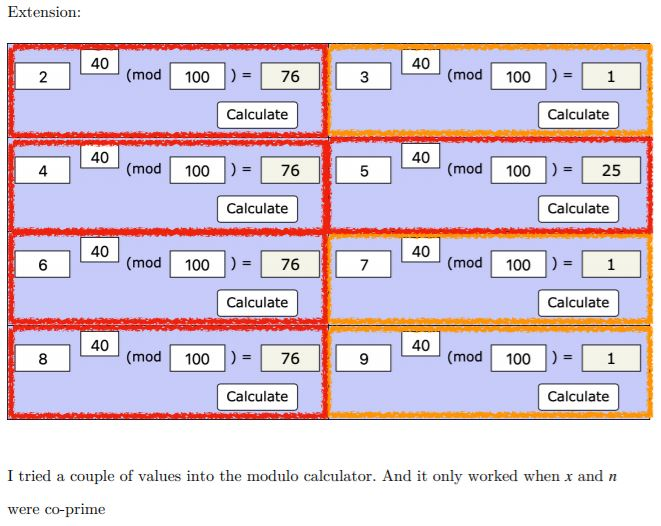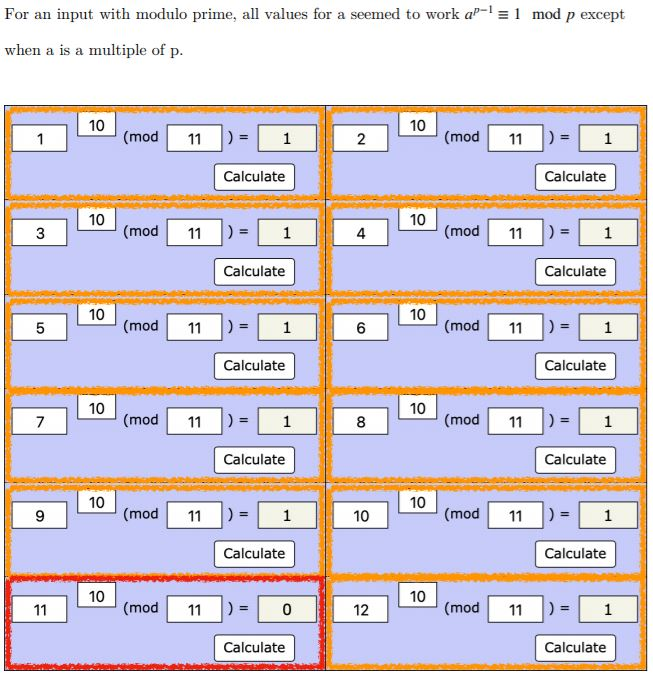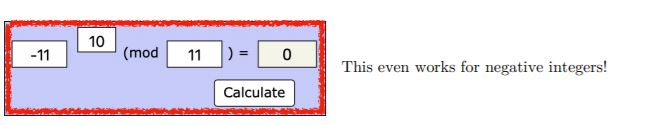Olivia and Wiktor proved it using similar arguments to each other. This is Olivia's proof, which uses ideas from More Adventures with Modular Arithmetic: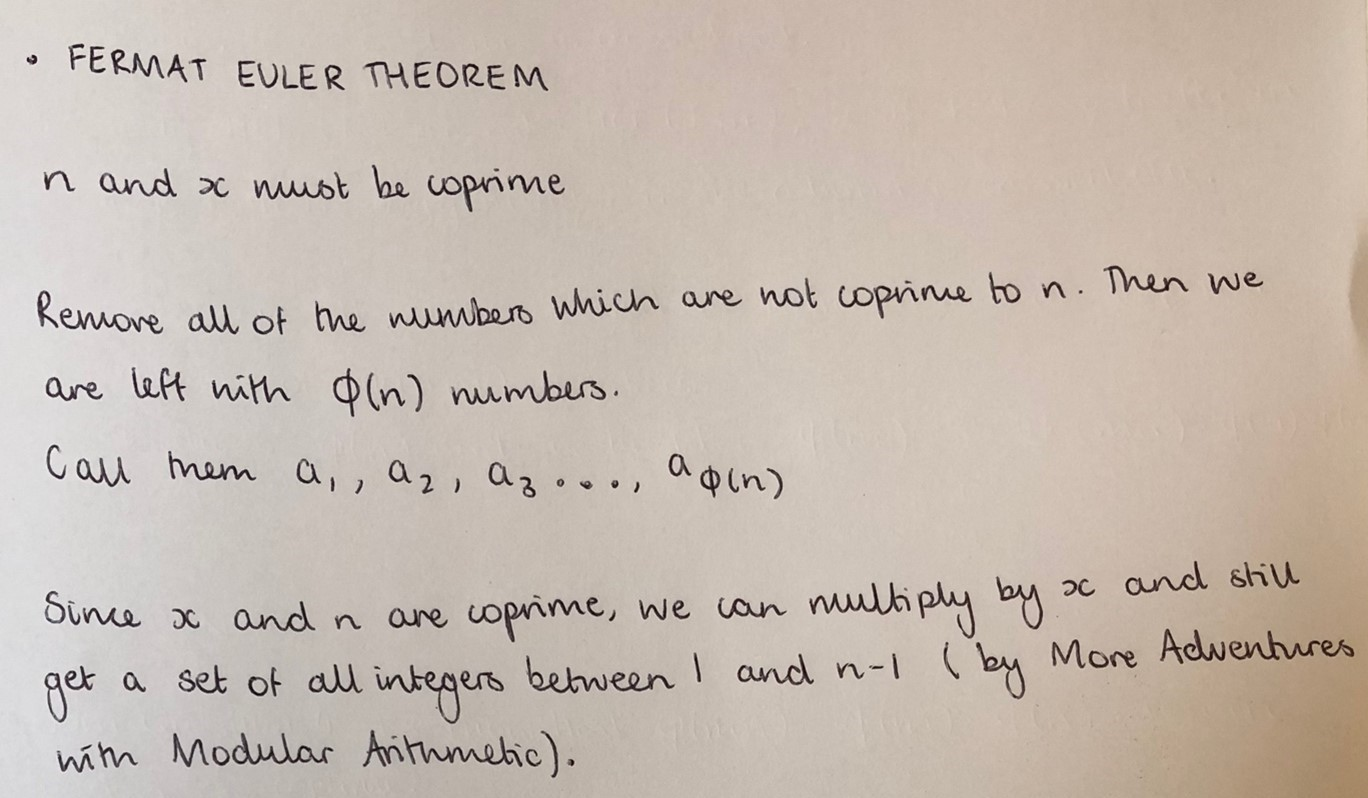Olivia means that when we multiply each of the numbers $a_1, a_2, a_3, ... , a_{\phi(n)}$ by $x,$ each of the products $a_1x, a_2x, a_3x, ... , a_{\phi(n)}x$ will be a different integer less than $n$ which is coprime to $n.$

Therefore $a_1x, a_2x, a_3x, ... , a_{\phi(n)}x$ and $a_1, a_2, a_3, ... , a_{\phi(n)}$ are the same numbers in some order.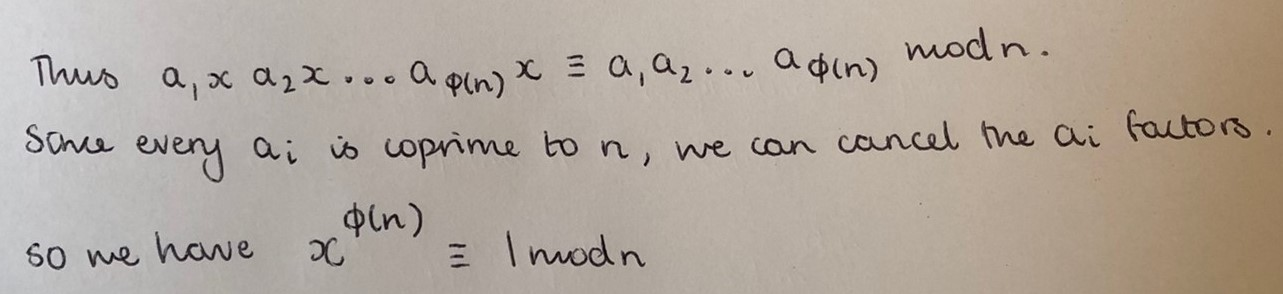Nayanika submitted two more videos which continues from the video at the top of the solution. In this video, Nayanika talks about the golden ratio and the Fibonacci numbers. Click here to see Nayanika's second video. Can you see how the rectangles in the image at the end are connected to the golden ratio?